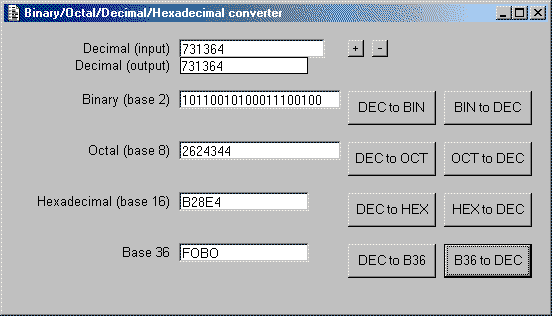# Tony Marston - 1st June 2002

There may be occasions when you want to convert a decimal number into another format, such as binary, octal or hexadecimal. You may even want to convert a number in one of these other formats back into decimal. Uniface does not contain any standard functions to do this conversion, so you have to write your own routines.

This document describes the pair of routines which I wrote to perform this conversion. One converts from DECIMAL to BASEn, the other converts from BASEn to DECIMAL. For those of you who slept through your maths lessons I will translate:

• base 2 = binary (uses only the digits 0 and 1)
• base 8 = octal (uses only the digits 0-7)
• base 10 = decimal (uses digits 0-9)
• base 16 = hexadecimal (uses digits 0-9 plus the letters A-F)
• base 36 = somethingdecimal (uses digits 0-9 plus the letters A-Z)

The form which I use to test these routines looks like this:The field labeled Decimal (input) is for the decimal number. You can then press one of the DEC to BIN/OCT/HEX/B36 buttons in order to convert that number into the chosen format. If you then press one of the BIN/OCT/HEX/B36 to DEC buttons this will convert that value back into decimal and place the result in the Decimal (output) field.

To convert from decimal into one of the other formats I use the following code:

```call DEC_TO_STRING(decimal_input, base, output_string)
```

where BASE is 2, 8, 16 or 36 (as explained above). The proc which is called looks like this:

```entry DEC_TO_STRING     ; convert decimal number to baseN
params
numeric pi_Decimal   : IN
numeric pi_Base      : IN
string  po_String    : OUT
endparams
variables
string  lv_Charset
numeric lv_Decimal
numeric lv_Remainder
string  lv_Char
endvariables

if (pi_Base < 2) | (pi_Base > 36) | (pi_Base = 10)
message "BASE must be in the range 2-9 or 11-36"
return(-1)
endif

; maximum character string is 36 characters
lv_Charset = "0123456789ABCDEFGHIJKLMNOPQRSTUVWXYZ"
; strip off excess characters (anything above pi_Base)
lv_Charset = lv_Charset[1,pi_Base]

lv_Decimal = pi_Decimal                      ; move to work area
repeat
; get remainder after dividing by BASE
lv_Remainder = (lv_Decimal % pi_Base)
lv_Remainder = lv_Remainder + 1           ; starts from 1, not zero

lv_Char      = lv_Charset[lv_Remainder:1] ; get CHAR from array
po_String    = "%%lv_Char%%po_String"     ; add to output

lv_Decimal   = lv_Decimal - (lv_Remainder -1)
lv_Decimal   = lv_Decimal / pi_Base

until (lv_Decimal < 1)

return(0)

end DEC_TO_STRING
```

To convert from one of the other formats back into decimal I use the following code:

```call STRING_TO_DEC(input_string, base, decimal_output)
```

where BASE must correspond to the format of INPUT_STRING. The proc which is called looks like this:

```entry STRING_TO_DEC     ; convert baseN to decimal number
params
string  pi_String    : IN
numeric pi_Base      : IN
numeric po_Decimal   : OUT
endparams
variables
string  lv_Charset
numeric lv_Base
string  lv_String
numeric lv_Remainder
string  lv_Char
endvariables

if (pi_Base < 2) | (pi_Base > 36) | (pi_Base = 10)
message "BASE must be in the range 2-9 or 11-36"
return(-1)
endif

; maximum character string is 36 characters
lv_Charset = "0123456789ABCDEFGHIJKLMNOPQRSTUVWXYZ"
; strip off excess characters (anything above pi_Base)
lv_Charset = lv_Charset[1,pi_Base]

lv_String  = pi_String          ; move to work area
repeat
lv_Char   = lv_String[1:1]   ; extract leading character
lv_String = lv_String     ; drop leading character

scan lv_Charset, lv_Char     ; get offset in Charset
if (\$result < 1)
message "Illegal character (%%lv_Char) in INPUT string"
return(-1)
endif

lv_Remainder = \$result -1    ; starts from zero, not 1

po_Decimal   = po_Decimal * pi_Base
po_Decimal   = po_Decimal + lv_Remainder

until (lv_String = "")

return(0)

end STRING_TO_DEC
```

As you can see the code in each of my routines is not too complex. Feel free to copy them and add them to your own application library.